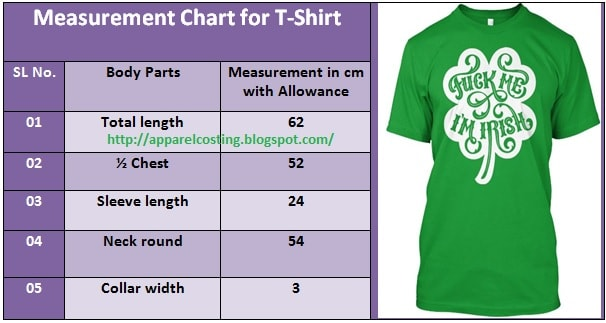# Apparel Costing

Solution of textile and apparel calculation

## How to Calculate Fabric Consumption for T-Shirt?

Knit Garments Consumption Calculation in Apparel Sector:
In apparel export order or business, fabrics consume the most part of the apparel costing amount. So, fabric consumption is very important factors for not only the export business but also for the apparel merchandisers. He must have to know the exact method for calculating this issue.

Knit Fabric Consumption Calculation Method for T-Shirt in Apparel Industry:
Calculate the fabric consumption for 10000 pcs “100% Cotton Single Jersey Round Neck T-Shirt” from the following measurement chart. Here, fabric G.S.M is 160Fig: Fabric consumption method for T-Shirt in apparel industry
Solution:

Apparel Weight Calculation or Fabric Consumption Calculation Formula:
To find out the apparel weight or fabric consumption, we should apply the following two formulas. By adding equation- (A) and equation (B), we will get the required fabric consumption for round neck t-shirt.

Fabric consumption calculation or apparel weight calculation,

[{S.L + T.L + (2 × S.A) + (2× H.A)} × (1/2 Chest + S.A)] × G.S.M × 2
= ……………………………………………………………………......………        ….. (A)
10000

And,

Round neck collar fabric consumption calculation,

{(Collar width + S.A) × (Neck round + S.A) × G.S.M × 2}
= …………………………………………....……………………             ……….. (B)
10000

Here,
S.A means Seam allowance,
T.L means Total length,
H.A means Hem allowance,
S.L means Sleeve length.

Apparel weight calculation for middle size i.e. L Size:

Let,
Hem allowance is 2cm,
Seam allowance is 2cm,

So,
From the equation- (A), we get,

{24+62+ (2×2) + (2×2)} × (52+2)] ×160×2
= ………………………………………………………..
10000

[{(24+62+4+4) × 54} × 160×2]
= ………………………………………….
10000

= 162.43 gms
= 0.162 kg

Again
From the equation- (B), we get,

{(3+2) × (54+2) ×160×2}
= ………………………………..
10000

5×56×160×2
= ………………………
10000

= 8.96 gms
= 0.09 kg

So,
Fabric consumption for one piece round neck T-shirt stands at,
= (0.162+0.09) kg
= 0.252 kg

And,
Fabric consumption for 10000 pcs round neck T-shirt is
= Total order quantity × fabric consumption for one piece t-shirt
= 10000 pcs × 0.252 kg
= 2520 kgs.

So,
To calculate the above apparel export order, it needs 2520 kgs 100% cotton single jersey fabrics.